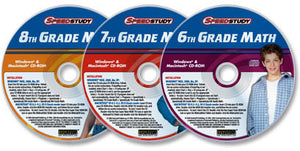## We will reopen in better times.# 3 CD-ROM Middle-School Math set (Speedstudy 6th, 7th, & 8th Grade Math)

• \$18.00
Unit price per

These programs are not just textbooks on CD-ROMs—they're like having a fun, personal tutor of your very own. These three Speedstudy Math programs provide a solid educational foundation designed to raise grades and test scores and improve math skills in the classroom and beyond. Using timed quizzes, step-by-step animations, and a fun 3-D interface, these products give students the tools they need to master important math concepts. Build basic math skills fast and painlessly, prepare for proficiency exams, and take the worry out of middle-school math. Each CD-ROM features curriculum-based lessons, designed by educators to help students understand and practice critical thinking and problem-solving skills.

6th Grade Math includes more than 40 standards-based lessons and 300+ interactive quiz questions covering factor trees, fractions, decimals, perimeter & area, percentages, measurement, and more.

Go a step further with 7th Grade Math. With more than 25 lessons and 200+ quiz questions, this program teaches such topics as integers, absolute values, negative fractions, ratios, exponents, graphing linear equations, scientific notation, and probability.

Prepare for high-school with 8th Grade Math's 25 lessons and 300+ quiz questions. Get a solid introduction to geometry, square roots, sequences and series, polynomial equations, the Pythagorean Theorem, and even trigonometry. In addition, each program includes an on-screen calculator, graphing calculator, links to online web help, and a searchable database of 500+ key terms.

These 3 CD-ROM products are Windows compatible (including XP, Vista, 7, 8 and 10).